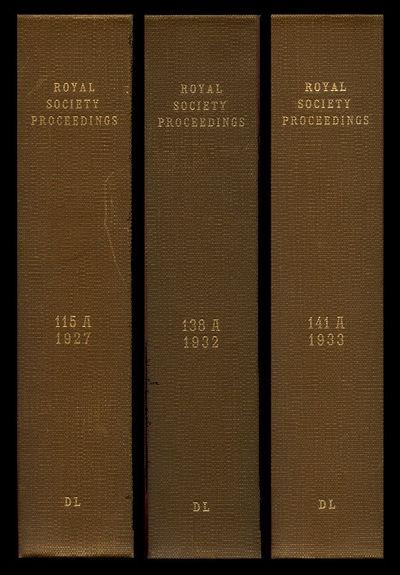Recently found by viaLibri....
2020-02-15 01:55:59Kermack, W. O. and A. G. McKendrick
A Contribution to the Mathematical Theory of Epidemics I in Proceedings of the Royal Society of London A 115, 1927) WITH Contributions to the Mathematical Theory of Epidemics. II. The Problem of Endemicity in Proceedings of the Royal Society of London A 138, 1932 WITH Contributions to the Mathematical Theory of Epidemics. III. Further Studies of the Problem of Endemicity in Proceedings of the Royal Society of London A 141, 1933
London: Harrison & Sons, Ltd, 2009. FIRST EDITION OF A THREE VOLUME LANDMARK SERIES OF PAPERS PROVIDING THE FIRST MATHEMATICAL ANALYSIS OF EPIDEMICS as well as "the first mathematical model that could be used to describe an influenza epidemic" (Coburn, "Modeling influenza," BMC Medicine, 7, 2009, 1741). This work is obviously particularly timeless in light of the Coronavirus COVID-19 outbreak. "Mathematical modeling of epidemics goes back to 1927 with the formulation of [these] classical equations" Lewis, Network Science, 8.1.1). The Kermack-McKendrick model is known as the Susceptible-Infections-Recovered (SIR) model and is used to depict the number of people infected with a contagious illness in a closed population over time. To simulate an influenza epidemic the model, Kermack and McKendrick developed the pioneering threshold theorem that enables the interval between epidemics and their size to be studied. "According to this, the introduction of infectious cases into a community of susceptibles would not give rise to an epidemic if the density of susceptibles was below a certain critical value. Once the critical value was exceeded, then there would be an epidemic of magnitude sufficient to reduce the density of susceptibles as far below the threshold as it originally was above" (Cliff, Deciphering Global Epidemics, 26). [Kermack and McKendrick's] SIR model has been used as a basis for all subsequent influenza models" (Lewis). Furthermore, beyond influenza models, "most modern mathematical epidemiology" is based on these classic papers (Watts, Six Degrees, 332). COND … [Click Below for Full Description]
Bookseller: Atticus Rare Books [United States]
Check availability: